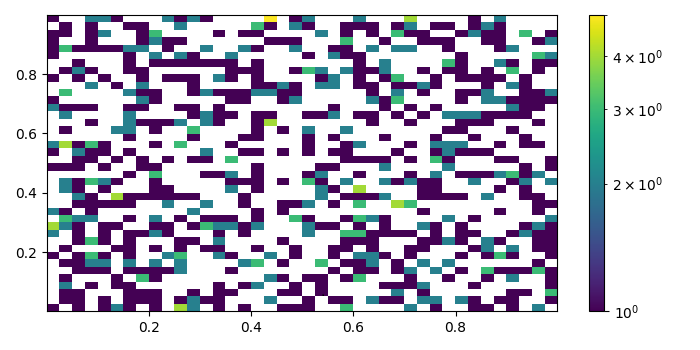# How do I use colorbar with hist2d in matplotlib.pyplot?

To use colorbar with hist2d in matplotlib.pyplot, we can take the following steps.

## Steps

• Set the figure size and adjust the padding between and around the subplots.

• Initialize a variable "N" for the number of sample data.

• Createx and y data points using numpy.

• Create a figure and a set of subplots using subplots() method.

• Make a 2D histogram plot using hist2D().

• Create a colorbar for the hist2d scalar mappable instance.

• To display the figure, use Show() method.

## Example

from matplotlib.colors import LogNorm
import matplotlib.pyplot as plt
import numpy as np

# Set the figure size
plt.rcParams["figure.figsize"] = [7.00, 3.50]
plt.rcParams["figure.autolayout"] = True

# Number of sample data
N = 1000

# Create x and y data points
x = np.random.rand(N)
y = np.random.rand(N)

fig, ax = plt.subplots()

# 2D histogram plot with x and y
hh = ax.hist2d(x, y, bins=40, norm=LogNorm())

fig.colorbar(hh, ax=ax)

# Display the plot
plt.show()


## Output

It will produce the following output −